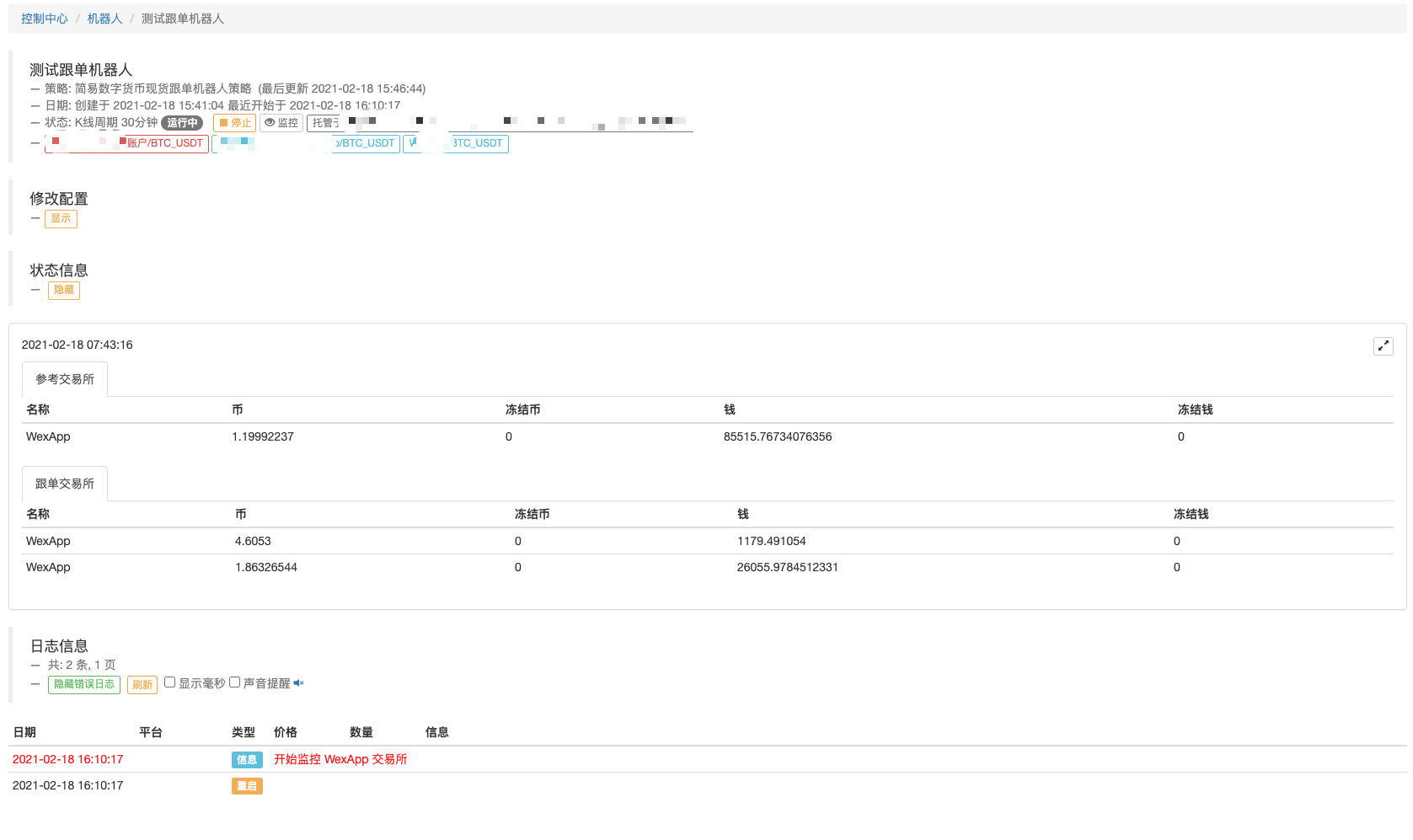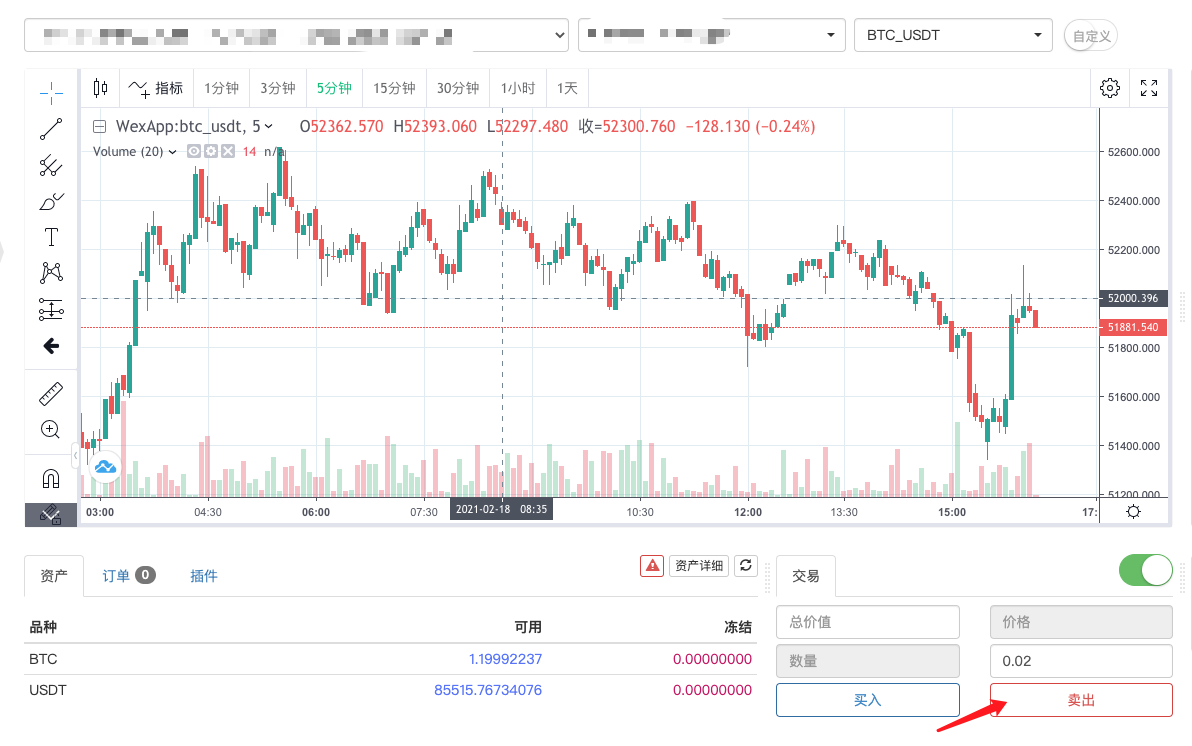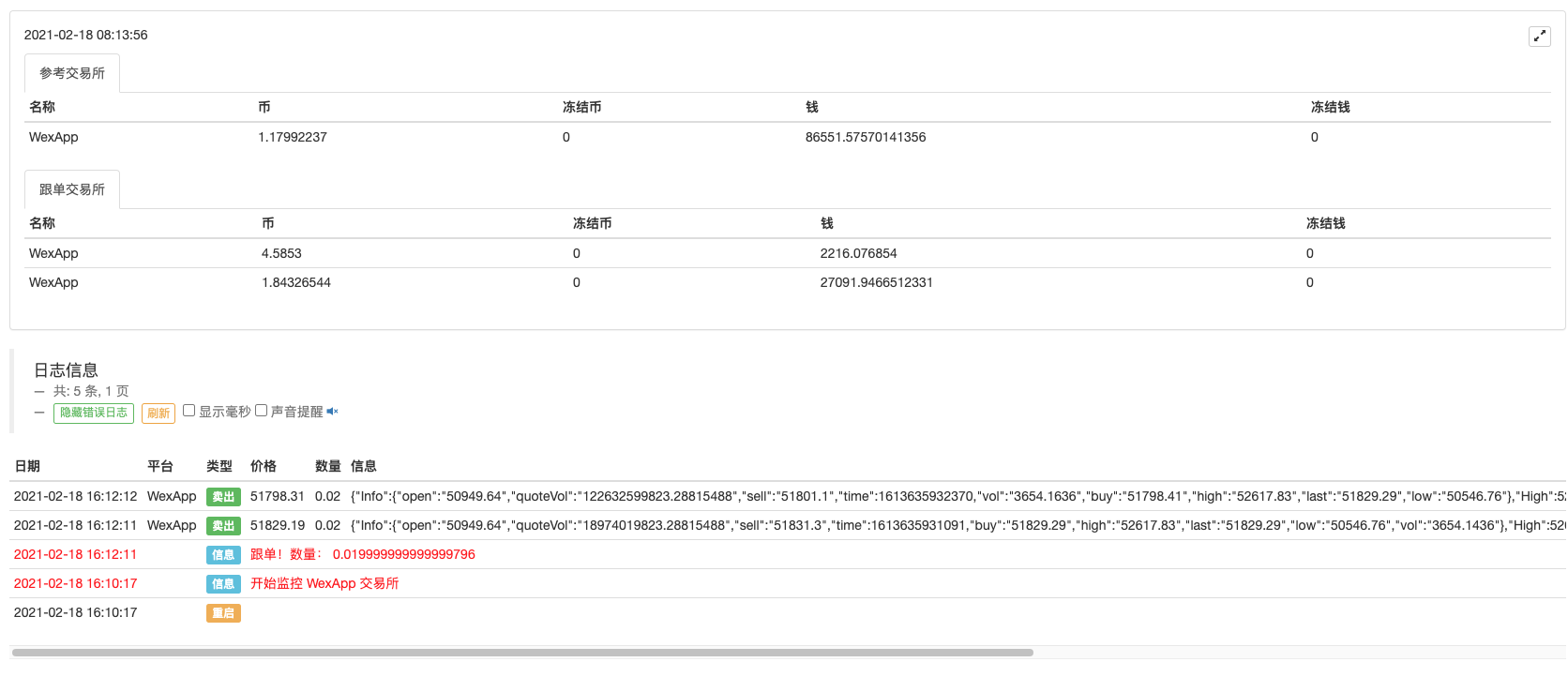# [原创] 实现一个简易数字货币现货跟单机器人

• 参考账户：被监控的账户，监控这个账户的一举一动。
• 跟单账户：跟单的账户，当参考账户发生动作，跟单账户执行同样的动作。

``````        // 检测跟单
var amount = (nowAcc.Stocks + nowAcc.FrozenStocks) - (initAcc.Stocks + initAcc.FrozenStocks)  // 检测币数发生的变化
var func = null
if (amount > 0) {   // 币变多了
func = \$.Buy    // 买入
} else if (amount < 0) {  // 币变少了
func = \$.Sell         // 卖出
} else {
continue
}

// 执行跟单
Log("跟单！数量：", Math.abs(amount), "#FF0000")
for (var i = 1 ; i < exchanges.length ; i++) {    // i 等于 0 时，是参考交易所，不处理，处理其它的跟单交易所
func(exchanges[i], Math.abs(amount))          // 执行具体的交易函数，可能是\$.Buy 或者是 \$.Sell ，具体看amount 是大于0，还是小于0
}

// 执行跟单之后更新参考交易所账户信息记录
initAcc = nowAcc                                  // 更新参考交易所最近一次的账户信息，用于下次对比
``````

``````function test() {
// 测试函数
var ts = new Date().getTime()
if (ts % (1000 * 60 * 60 * 6) > 1000 * 60 * 60 * 5.5) {
Sleep(1000 * 60 * 10)
var x = Math.random()
if (x > 0.5) {
\$.Buy(exchange, x / 10)
} else {
\$.Sell(exchange, x / 10)
}
}
}

function main() {
LogReset(1)
if (exchanges.length < 2) {
throw "没有跟单的交易所"
}
var exName = exchange.GetName()
// 检测参考交易所
if (exName.includes("Futures_")) {
throw "仅支持现货跟单"
}
Log("开始监控", exName, "交易所", "#FF0000")

// 检测跟单交易所
for (var i = 1 ; i < exchanges.length ; i++) {
if (exchanges[i].GetName().includes("Futures_")) {
throw "不支持期货交易所跟单"
}
}

var initAcc = _C(exchange.GetAccount)
while(1) {
if(IsVirtual()) {
// 测试函数
test()
}
Sleep(5000)

// 更新参考账户当前的账户信息
var nowAcc = _C(exchange.GetAccount)

// 参考交易所账户信息
var refTbl = {
type : "table",
title : "参考交易所",
cols : ["名称", "币", "冻结币", "钱", "冻结钱"],
rows : []
}
refTbl.rows.push([exName, nowAcc.Stocks, nowAcc.FrozenStocks, nowAcc.Balance, nowAcc.FrozenBalance])

// 跟单交易所账户信息
var followTbl = {
type : "table",
title : "跟单交易所",
cols : ["名称", "币", "冻结币", "钱", "冻结钱"],
rows : []
}
for (var i = 1 ; i < exchanges.length ; i++) {
var acc = _C(exchanges[i].GetAccount)
var name = exchanges[i].GetName()
followTbl.rows.push([name, acc.Stocks, acc.FrozenStocks, acc.Balance, acc.FrozenBalance])
}

// 状态栏显示
LogStatus(_D(), "\n`" + JSON.stringify(refTbl) + "`", "\n`" + JSON.stringify(followTbl) + "`")

// 检测跟单
var amount = (nowAcc.Stocks + nowAcc.FrozenStocks) - (initAcc.Stocks + initAcc.FrozenStocks)
var func = null
if (amount > 0) {
func = \$.Buy
} else if (amount < 0) {
func = \$.Sell
} else {
continue
}

// 执行跟单
Log("跟单！数量：", Math.abs(amount), "#FF0000")
for (var i = 1 ; i < exchanges.length ; i++) {
func(exchanges[i], Math.abs(amount))
}

// 执行跟单之后更新参考交易所账户信息记录
initAcc = nowAcc
}
}
``````##### 相关下载
###### 登录后评论
Copyright © 2019 宽客在线